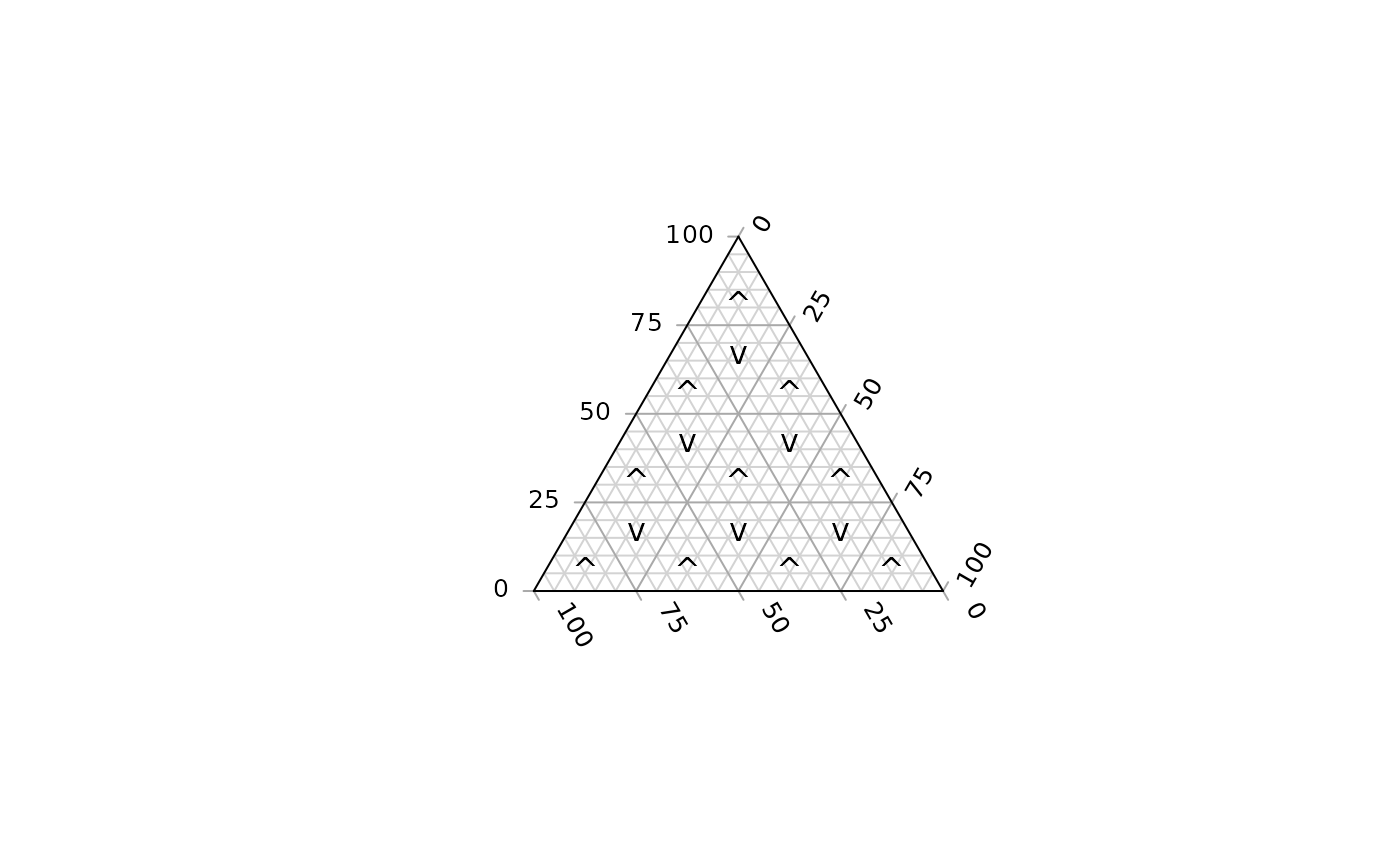Calculate x and y coordinates of the midpoints of triangles tiled to cover a ternary plot.

TriangleCentres(resolution = 48L, direction = getOption("ternDirection", 1L))

## Arguments

resolution

The number of triangles whose base should lie on the longest axis of the triangle. Higher numbers will result in smaller subdivisions and smoother colour gradients, but at a computational cost.

direction

(optional) Integer specifying the direction that the current ternary plot should point: 1, up; 2, right; 3, down; 4, left.

## Value

TriangleCentres() returns a matrix with three named rows:

• x x coordinates of triangle midpoints;

• y y coordinates of triangle midpoints;

• triDown 0 for upwards-pointing triangles, 1 for downwards-pointing.

Add triangles to a plot: TernaryTiles()

Other coordinate translation functions: ReflectedEquivalents(), TernaryCoords(), XYToTernary()

Other tiling functions: Polygon-Geometry, TriangleInHull()

## Examples

TernaryPlot(grid.lines = 4)
centres <- TriangleCentres(4)
text(centres["x", ], centres["y", ], ifelse(centres["triDown", ], "v", "^"))